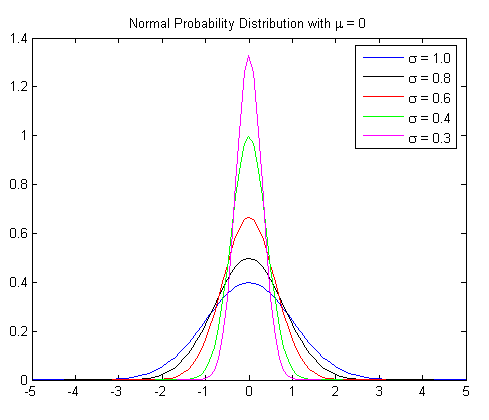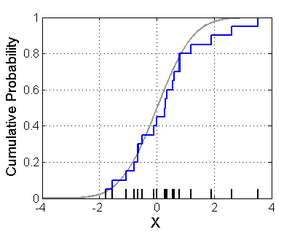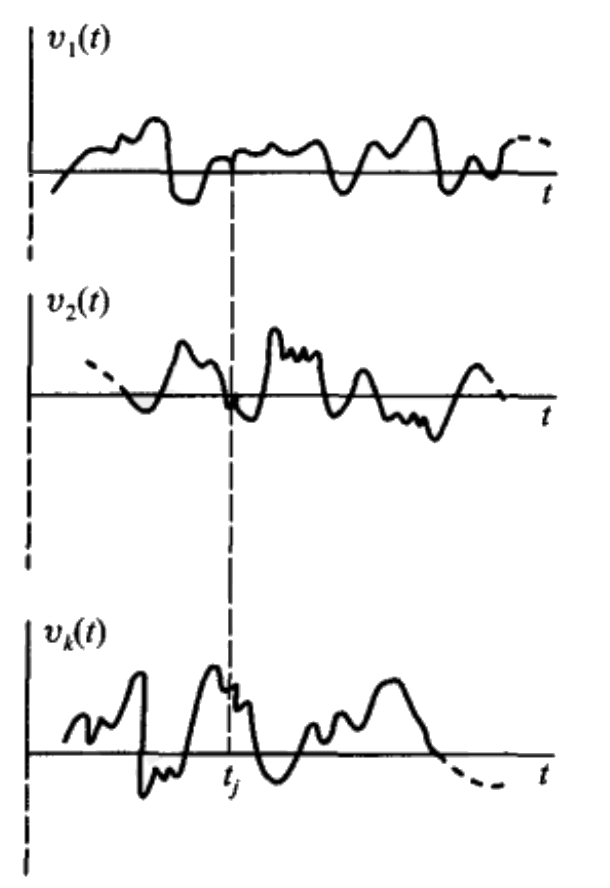# Probability Theory

## 1 概率论简介

Probability theory is the branch of mathematics concerned with probability, the analysis of random phenomena.

### 1.1 Laplace评概率论

Probability theory is nothing but common sense reduced to calculation. – by Laplace

### 1.2 随机试验、样本空间、事件

1. 可以在相同的条件下重复地进行；
2. 每次试验的可能结果不止一个，并且能事先知道试验的所有可能结果（这些所有可能的结果所组成的集合称为 样本空间 ）；
3. 进行一次试验之前不能确定哪一个结果会出现。

“连续掷一个硬币两次，正反面的出现情况”是一个随机试验。样本空间可表示为： $$S=\{HH, HT, TH, TT \}$$ ， $$H$$ 表示正面， $$T$$ 表示反面。“两次出现同一面”是一个事件，可表示为： $$A=\{ HH, TT\}$$ 。

### 1.3 什么是概率

1933年，俄国数学家 Andrei N. Kolmogorov 建立了概率论的公理化体 (Probability axioms)，给出了“概率”的严格定义。

1. 非负性：对于每一个事件 $$A$$ ，有 $$P(A) \ge 0$$
2. 规范性：对于必然事件 $$S$$ ，有 $$P(S) =1$$
3. 可列可加性：设 $$A_{1}, A_{2}, \cdots$$ 是两两互不相容的事件，即对于 $$A_{i}A_{i} = \emptyset, i \ne j$$ ，有 $$P(A_1 \cup A_2 \cup \cdots) = P(A_1) + P(A_2) + \cdots$$

### 1.4 条件概率

$P(B|A) = \frac{P(AB)}{P(A)}$

#### 1.4.1 Product Rule（即乘法公式）

$P(AB) = P(B|A)P(A)$

$P(ABC) = P(C|AB)P(B|A)P(A)$

#### 1.4.2 Law of Total Probability（即全概率公式）

(1) $$B_i B_j = \emptyset, i \neq j, i,j = 1,2,\cdots, n$$
(2) $$B_1 \cup B_2 \cup \cdots \cup B_n = S$$

\begin{aligned} P(A) &= P(A|B_1)P(B_1) + P(A|B_2)P(B_2) + \cdots + P(A|B_n)P(B_n) \\ &= \sum_{i=1}^{n} P(A|B_i)P(B_i) \end{aligned}

##### 1.4.2.1 Law of Total Probability（连续型随机变量的情况）

\begin{aligned}f_X(x) & = \int_{y} f(x,y) \, \mathrm{d}y \\ & = \int_{y} f_{X|Y}(x \mid y) \cdot f_Y(y) \, \mathrm{d}y \end{aligned}

#### 1.4.3 贝叶斯公式

$P(B|A) = \frac{P(A|B)P(B)}{P(A)}$

$P(B_i|A) = \frac{P(A|B_i) P(B_i)}{\sum_{j=1}^{n} P(A|B_j)P(B_j)}$

##### 1.4.3.1 贝叶斯公式应用实例

$P(B|A) = \frac{0.98 \times 0.95}{0.98 \times 0.95 + 0.55 \times 0.05} = 0.97$

##### 1.4.3.2 再看“贝叶斯公式”

$P(B|A) = \frac{P(A|B)}{P(A)} P(B)$

$\text{后验概率} = \text{标准似然度} * \text{先验概率}$

#### 1.4.4 用贝叶斯公式推测“事件的主要原因”

\begin{aligned} P(B_i|A) &= \frac{P(A|B_i) P(B_i)}{P(A)} \\ & \propto P(A|B_i) P(B_i) \end{aligned}

### 1.5 随机事件的独立性

$P(AB) = P(A)P(B)$

\begin{aligned}P(A \mid B) &= \frac{P(AB)}{P(B)} \\ &= \frac{P(A)P(B)}{P(B)} \\ &= P(A) \end{aligned}

## 2 随机变量及其分布

### 2.2 随机变量的分布函数(Cumulative distribution function, CDF)

$F(x)=P\{X \le x\}, -\infty < x < \infty$

1. 非降性。如果 $$x_{1} < x_{2}$$ ，则 $$F(x_{1}) \le F(x_{2})$$
2. 有界性。 $$0 \le F(x) \le 1$$ 且 $$F(-\infty)=0, F(\infty) =1$$
3. 右连续性。 $$F(x) = F(x+0)$$

### 2.3 离散型随机变量及其“概率质量函数”(Probability mess function, pmf)

1. $$p_{k} \ge 0$$
2. $$\sum_{k=1}^{\infty}p_{k} = 1$$

#### 2.3.1 (0-1)分布（又称Bernoulli distribution）

$P\{X=k\} = p^{k}(1-p)^{1-k}, k=0,1 \quad (0 < p < 1)$

(0-1)分布的分布律也可写为：
$\begin{array}{c|cc} X & 0 & 1 \\ \hline p_{k} & 1-p & p \\ \end{array}$

#### 2.3.2 二项分布（它是(0-1)分布的更通用形式）

$P\{X=k\} = {n \choose k} p^{k}(1-p)^{n-k}, k = 0,1,2, \cdots, n.$

#### 2.3.3 泊松分布(Poisson distribution)

$P\{X=k\} = \frac{\lambda^{k}e^{-\lambda}}{k!}, k=0,1,2, \cdots \quad (\lambda > 0)$

### 2.4 连续型随机变量及其“概率密度函数”(Probability density function, pdf)

$F(x) = \int_{-\infty}^{x}f(t)dt$

1. $$f(x) \ge 0$$
2. $$\int_{-\infty}^{\infty}f(x)dx = 1$$
3. 对于任意实数 $$x_{1},x_{2} \; (x_{1} \le x_{2})$$ 有： $$P\{x_{1} < X \le x_{2}\} = F(x_{2}) - F(x_{1}) = \int_{x_{1}}^{x_{2}}f(x)dx$$
4. 若 $$f(x)$$ 在点 $$x$$ 处连续，则有 $$F'(x) = f(x)$$

#### 2.4.1 均匀分布

$f(x)= \begin{cases} \frac{1}{b-a} & a \le x \le b \\ 0 & \text{otherwise} \end{cases}$

#### 2.4.2 指数分布

$f(x)= \begin{cases} \lambda e^{-\lambda x} & x \ge 0 \\ 0 & \text{otherwise} \end{cases}$

##### 2.4.2.1 指数分布重要特点——无记忆性

$P\{X > s +t \; | \; X > s\} = P\{X > t\}$

#### 2.4.3 正态分布（又称高斯分布）

$f(x) = \frac{1}{\sqrt{2\pi}\sigma}e^{-\frac{(x-\mu)^{2}}{2\sigma^{2}}} \quad (-\infty < x < \infty)$Figure 1: 正态分布概率密度（摘自：https://explorable.com/normal-probability-distribution

$\phi(x)=\frac{1}{\sqrt{2\pi}}e^{-\frac{x^{2}}{2}}$

### 2.5 随机变量的函数的分布

\begin{aligned} F_Y(y) &= P\{ Y \le y \} \\ &= P\{ X^2 \le y \} \\ &= P\{ - \sqrt{y} \le X \le \sqrt{y} \} \\ &= F_X(\sqrt{y}) - F_X(- \sqrt{y}) \\ \end{aligned}

$f_Y(y) = \begin{cases} \frac{1}{2\sqrt{y}} \left[f_X(\sqrt{y}) + f_X(-\sqrt{y}) \right], & y>0 \\ 0, & y \le 0 \end{cases}$

## 3 多维随机变量及其分布

### 3.1 联合分布

$F(x,y) = P\{(X \le x) \cap (Y \le y)\} \stackrel{\text{记为}}{=} P\{ X \le x, Y \le y \}$

#### 3.1.1 二维离散型随机变量的联合分布律

$p_{ij} \ge 0, \; \sum_{i=1}^{\infty}\sum_{j=1}^{\infty} p_{ij} = 1$

$\begin{array}{c|ccccc} Y \backslash X & x_1 & x_2 & \cdots & x_i & \cdots \\ \hline y_1 & p_{11} & p_{21} & \cdots & p_{i1} & \cdots \\ y_2 & p_{12} & p_{22} & \cdots & p_{i2} & \cdots \\ \vdots & \vdots & \vdots & & \vdots & \\ y_j & p_{1j} & p_{2j} & \cdots & p_{ij} & \cdots \\ \vdots & \vdots & \vdots & & \vdots & \\ \end{array}$

$F(x,y) = \sum_{x_i \le x}\sum_{y_j \le y} p_{ij}$

#### 3.1.2 二维连续型随机变量的联合概率密度

$F(x,y) = \int_{-\infty}^y \int_{-\infty}^{x} f(u,v) \,\mathrm{d}u\mathrm{d}v$

### 3.2 边缘分布

$F_X(x) = P\{X \le x \} = P\{X \le x, Y < \infty \} = F(x,\infty)$

$F_Y(y) = F(\infty, y)$

#### 3.2.1 二维离散型随机变量的边缘分布律（“边缘”名字由来）

$p_{i \cdot} = \sum_{j=1}^{\infty}p_{ij} = P\{X=x_i\}, \; i = 1,2,\cdots$

$p_{\cdot j} = \sum_{i=1}^{\infty}p_{ij} = P\{Y=y_i\}, \; y = 1,2,\cdots$

$\begin{array}{c|cccc|c} Y \backslash X & 1 & 2 & 3 & 4 & p_{\cdot j}=P\{ Y=j \} \\ \hline 1 & \frac{1}{4} & \frac{1}{8} & \frac{1}{12} & \frac{1}{16} & \frac{25}{48} \\ 2 & 0 & \frac{1}{8} & \frac{1}{12} & \frac{1}{16} & \frac{13}{48} \\ 3 & 0 & 0 & \frac{1}{12} & \frac{1}{16} & \frac{7}{48} \\ 4 & 0 & 0 & 0 & \frac{1}{16} & \frac{1}{16} \\ \hline p_{i \cdot} = P\{ X = i \} & \frac{1}{4} & \frac{1}{4} & \frac{1}{4} & \frac{1}{4} & 1 \\ \end{array}$

#### 3.2.2 二维连续型随机变量的边缘概率密度

$f_X(x) = \int_{-\infty}^{\infty} f(x,y) \, \mathrm{d}y = \int_{y} f(x,y) \, \mathrm{d}y$

$f_Y(y) = \int_{-\infty}^{\infty} f(x,y) \, \mathrm{d}x = \int_{x} f(x,y) \, \mathrm{d}x$

### 3.3 条件分布

#### 3.3.1 二维离散型随机变量的条件分布律

$P\{X=x_i \mid Y=y_j\} = \frac{P\{X=x_i , Y=y_j\}}{P\{Y=y_j\}} = \frac{p_{ij}}{p_{\cdot j}}, \; i = 1,2, \cdots$

$P\{Y=y_i \mid X=x_i\} = \frac{P\{X=x_i , Y=y_j\}}{P\{X=x_i\}} = \frac{p_{ij}}{p_{i \cdot}}, \; j = 1,2, \cdots$

#### 3.3.2 二维连续型随机变量的条件概率密度

$f_{X|Y}(x \mid y) = \frac{f(x,y)}{f_Y(y)}$

$f_{Y|X}(y \mid x) = \frac{f(x,y)}{f_X(x)}$

### 3.4 相互独立的随机变量

$P\{X \le x, Y \le y\} = P\{X \le x\} P\{Y \le y\}$

#### 3.4.1 离散型随机变量相互独立

$P\{X=x_i, Y=y_j\} = P\{X=x_i\} P\{Y=y_j\}$

#### 3.4.2 连续型随机变量相互独立

$f(x,y) = f_X(x) f_Y(y)$

### 3.5 两个随机变量的函数的分布

#### 3.5.1 Z=X+Y的分布

$f_{X+Y}(z) = \int_{- \infty}^{\infty} f(z-y,y) \, \mathrm{d} y$

$f_{X+Y}(z) = \int_{- \infty}^{\infty} f(x,z-x) \, \mathrm{d} x$

$f_{X+Y}(z) = \int_{- \infty}^{\infty} f_X(z-y)f_Y(y) \, \mathrm{d} y$

$f_{X+Y}(z) = \int_{- \infty}^{\infty} f_X(x)f_Y(z-x) \, \mathrm{d} x$

$\begin{gathered} f_X(x) = \frac{1}{\sqrt{2\pi}} e^{-x^2/2}, \quad -\infty < x < \infty \\ f_Y(y) = \frac{1}{\sqrt{2\pi}} e^{-y^2/2}, \quad -\infty < y < \infty \end{gathered}$

\begin{aligned} f_Z(z) &= \int_{- \infty}^{\infty} f_X(x)f_Y(z-x) \, \mathrm{d} x \\ &= \frac{1}{2\pi} \int_{- \infty}^{\infty} e^{-\frac{x^2}{2}} \cdot e^{-\frac{(z-x)^2}{2}} \, \mathrm{d} x \\ &= \frac{1}{2\pi} e^{-\frac{z^2}{4}} \int_{- \infty}^{\infty} e^{-(x- \frac{z}{2})^2} \, \mathrm{d} x \\ \end{aligned}

$f_Z(z) = \frac{1}{2\pi} e^{-\frac{z^2}{4}} \int_{- \infty}^{\infty} e^{-t^2} \, \mathrm{d} t = \frac{1}{2\pi} e^{-\frac{z^2}{4}} \sqrt{\pi} = \frac{1}{2\sqrt{\pi}} e^{-\frac{z^2}{4}}$

#### 3.5.2 Z=Y/X和Z=XY的分布

\begin{aligned} f_{Y/X}(z) & = \int_{- \infty}^{\infty} \vert x \vert \, f(x,xz) \, \mathrm{d} x \\ f_{XY}(z) & = \int_{- \infty}^{\infty} \frac{1}{\vert x \vert} \, f(x,\frac{z}{x}) \, \mathrm{d} x \end{aligned}

\begin{aligned} f_{Y/X}(z) & = \int_{- \infty}^{\infty} \vert x \vert \, f_X(x)f_Y(xz) \, \mathrm{d} x \\ f_{XY}(z) & = \int_{- \infty}^{\infty} \frac{1}{\vert x \vert} \, f_X(x)f_Y(\frac{z}{x}) \, \mathrm{d} x \end{aligned}

## 4 随机变量的数字特征

### 4.1 数学期望(Expectation)

$E(X)=\sum_{i}^{\infty}x_{k}p_{k}$

$E(X)=\int_{-\infty}^{\infty} xf(x)dx$

(1) 设 $$C$$ 是常数，则 $$E(C)=C$$ 。
(2) 设 $$X$$ 是随机变量， $$C$$ 是常数，则：
$E(CX) = C E(X)$
(3) 设 $$X,Y$$ 是两个随机变量，则：
$E(X+Y) = E(X) + E(Y)$
(4) 如果 $$X,Y$$ 是相互独立的随机变量，则：
$E(XY) = E(X) E(Y)$

#### 4.1.1 随机变量函数的期望

(1) 如果 $$X$$ 是离散型随机变量，它的分布律为 $$P \{ X=x_k \} = p_k, k=1,2,\cdots$$ ，如果 $$\sum_{k=1}^{\infty}g(x_k)p_k$$ 绝对收敛，则：
$E(Y) = E[g(X)] = \sum_{k=1}^{\infty}g(x_k)p_k$
(2) 如果 $$X$$ 是连续型随机变量，它的概率密码为 $$f(x)$$ ，如果 $$\int_{- \infty}^{\infty}g(x)f(x) \, \mathrm{d}x$$ 绝对收敛，则：
$E(Y) = E[g(X)] = \int_{- \infty}^{\infty}g(x)f(x) \, \mathrm{d}x$

### 4.2 方差(Variance)和标准差(Standard deviation)

$D(X)=Var(X)=E[(X-E(X))^2]$

$D(X) = \sum_{k=1}^{\infty}[x_k - E(X)]^2 p_k$

$D(X) = \int_{- \infty}^{\infty}[x - E(X)]^2 f(x) dx$

$\sigma(X)=\sqrt{D(X)}=\sqrt{Var(X)}$

(1) 设 $$C$$ 是常数，则 $$D(C)=0$$ 。
(2) 设 $$X$$ 是随机变量， $$C$$ 是常数，则：
\begin{aligned} D(CX) = C^2 D(X) \\ D(X+C) = D(X) \end{aligned}
(3) 设 $$X,Y$$ 是两个随机变量，则：
$D(X+Y) = D(X) + D(Y) + 2 E\{ (X-E(X)) (Y-E(Y)) \}$

$D(X+Y) = D(X) + D(Y)$

### 4.3 协方差(Covariance)与相关系数(Correlation coefficient)

$Cov(X,Y) = E[(X-E(X))(Y-E(Y))]$

$\rho_{XY} = \frac{Cov(X,Y)}{\sigma(X)\sigma(Y)}=\frac{Cov(X,Y)}{\sqrt{Var(X)}\sqrt{Var(Y)}}$

### 4.4 协方差矩阵(Covariance matrix)

$C=\left( \begin{array}{cc} Cov(X,X) & Cov(X,Y) \\ Cov(Y,X) & Cov(Y,Y) \end{array} \right)=\left( \begin{array}{cc} Var(X) & Cov(X,Y) \\ Cov(X,Y) & Var(Y) \end{array} \right)$

$C=\left( \begin{array}{cccc} Cov(X_1,X_1) & Cov(X_1,X_2) & \cdots & Cov(X_1, X_n)\\ Cov(X_2,X_1) & Cov(X_2,X_2) & \cdots & Cov(X_2, X_n)\\ \vdots & \vdots & &\vdots \\ Cov(X_n,X_1) & Cov(X_n,X_2) & \cdots & Cov(X_n, X_n)\\ \end{array} \right)$

#### 4.4.1 n维正态随机变量的概率密度

$f(x_1, x_2) = \frac{1}{2\pi \sigma_1 \sigma_2 \sqrt{1-\rho^2}} \exp \left\{ - \frac{1}{2(1-\rho^2)} \left[\frac{(x_1 - \mu_1)^2}{\sigma_1} -2\rho \frac{(x_1 - \mu_1)(x_2 - \mu_2)}{\sigma_1 \sigma_2} + \frac{(x_2 - \mu_2)^2}{\sigma_2} \right] \right\}$

$\boldsymbol{x} = \begin{bmatrix} x_1 \\ x_2 \end{bmatrix}, \; \boldsymbol{\mu} = \begin{bmatrix} \mu_1 \\ \mu_2 \end{bmatrix}$
$$(X_1, X_2)$$ 的协方差矩阵（其中矩阵元素 $$c_{ij} = Cov(X_i, X_j)$$ ）为：
$\Sigma = \begin{bmatrix} c_{11} & c_{12} \\ c_{21} & c_{22} \end{bmatrix} = \begin{bmatrix} \sigma_2^2 & \rho \sigma_1 \sigma_2 \\ \rho \sigma_1 \sigma_2 & \sigma_1^2 \end{bmatrix}$

$\Sigma^{-1} = \frac{1}{\det \Sigma} \begin{bmatrix} \sigma_2^2 & - \rho \sigma_1 \sigma_2 \\ - \rho \sigma_1 \sigma_2 & \sigma_1^2 \end{bmatrix}$

\begin{aligned} (\boldsymbol{x} - \boldsymbol{\mu})^{\mathsf{T}} \Sigma^{-1} (\boldsymbol{x} - \boldsymbol{\mu}) & = \frac{1}{\det \Sigma} \begin{bmatrix} x_1 - \mu_1 & x_2 - \mu_2 \end{bmatrix} \begin{bmatrix} \sigma_2^2 & - \rho \sigma_1 \sigma_2 \\ - \rho \sigma_1 \sigma_2 & \sigma_1^2 \end{bmatrix} \begin{bmatrix} x_1 - \mu_1 \\ x_2 - \mu_2 \end{bmatrix} \\ \\ & = \frac{1}{1-\rho^2} \left[\frac{(x_1 - \mu_1)^2}{\sigma_1} -2\rho \frac{(x_1 - \mu_1)(x_2 - \mu_2)}{\sigma_1 \sigma_2} + \frac{(x_2 - \mu_2)^2}{\sigma_2} \right] \end{aligned}

$f(x_1, x_2) = \frac{1}{(2\pi)^{2/2} (\det \Sigma)^{1/2}} \exp \left\{ - \frac{1}{2} (\boldsymbol{x} - \boldsymbol{\mu})^{\mathsf{T}} \Sigma^{-1} (\boldsymbol{x} - \boldsymbol{\mu}) \right\}$

##### 4.4.1.1 n维正态随机变量及其性质

$\boldsymbol{x} = \begin{bmatrix} x_1 \\ x_2 \\ \vdots \\ x_n \end{bmatrix}, \; \boldsymbol{\mu} = \begin{bmatrix} \mu_1 \\ \mu_2 \\ \vdots \\ \mu_n \end{bmatrix} = \begin{bmatrix} E(X_1) \\ E(X_2) \\ \vdots \\ E(X_n) \end{bmatrix}$
$$n$$ 维正态随机变量 $$(X_1,X_2, \cdots, X_n)$$ 的概率密度的定义为：
\begin{aligned} f(x_1,x_2, \cdots, x_n) &= \frac{1}{(2\pi)^{n/2} (\det \Sigma)^{1/2}} \exp \left\{ - \frac{1}{2} (\boldsymbol{x} - \boldsymbol{\mu})^{\mathsf{T}} \Sigma^{-1} (\boldsymbol{x} - \boldsymbol{\mu}) \right\} \\ &= \det(2\pi \Sigma)^{-\frac{1}{2}} \exp \left\{ - \frac{1}{2} (\boldsymbol{x} - \boldsymbol{\mu})^{\mathsf{T}} \Sigma^{-1} (\boldsymbol{x} - \boldsymbol{\mu}) \right\} \\ \end{aligned}

$$n$$ 维正态随机变量具有以下四条重要性质（这里不证明）：
(1) $$n$$ 维正态随机变量 $$(X_1,X_2, \cdots, X_n)$$ 的每一个分量 $$X_i, i=1,2,\cdots,n$$ 都是正态随机变量；反之，若 $$X_i, i=1,2,\cdots,n$$ 都是正态随机变量，且相互独立，则 $$(X_1,X_2, \cdots, X_n)$$ 是 $$n$$ 维正态随机变量。
(2) $$n$$ 维随机变量 $$(X_1,X_2, \cdots, X_n)$$ 服从 $$n$$ 维正态分布的充要条件是 $$X_1,X_2, \cdots, X_n$$ 的任意的线性组合：
$l_1X_1 + l_2X_2 + \cdots + l_n X_n$

(3) 若 $$(X_1,X_2, \cdots, X_n)$$ 服从 $$n$$ 维正态分布，设 $$Y_1,Y_2, \cdots, Y_m$$ 分别是 $$X_1,X_2, \cdots, X_n$$ 的非零线性组合，则 $$(Y_1,Y_2, \cdots, Y_m)$$ 是 $$m$$ 维正态分布随机变量。
(4) 设 $$(X_1,X_2, \cdots, X_n)$$ 服从 $$n$$ 维正态分布，则 “ $$X_1,X_2, \cdots, X_n$$ 相互独立”和“ $$X_1,X_2, \cdots, X_n$$ 两两不相关”是等价的。

### 4.5 矩(Moment)和矩生成函数(Moment-generating function)

#### 4.5.1 矩(Moment)

$E(X^k), \quad k=1,2,\cdots$

$E[(X - E(X))^k ], \quad k=1,2,\cdots$

##### 4.5.1.1 标准矩(Standardized moment)、偏度(Skewness)和峰度(Kurtosis)

$\frac{\mu_k}{\sigma^k}$

$\frac{\mu_k}{\sigma^k} = \frac{E[(X - E(X))^k ]}{\left( E[(X - E(X))^2] \right)^{k/2}}$

#### 4.5.2 矩生成函数(Moment-generating function)

$M_X(t) := E \left[ e^{tX}\right] = \int_{-\infty}^{\infty} e^{tx} f(x) \, \mathrm{d} x, \qquad t \in \mathbb{R}$

$e^{tX} = 1 + tX + \frac{t^2 X^2}{2!} + \frac{t^3 X^3}{3!} + \cdots + \frac{t^n X^n}{n!} + \cdots$

\begin{aligned} M_X(t) = E \left[ e^{tX}\right] & = 1 + t E(X) + \frac{t^2 E(X^2)}{2!} + \frac{t^3 E(X^3)}{3!} + \cdots + \frac{t^n E(X^n)}{n!} + \cdots \\ & = 1 + tm_1 + \frac{t^2 m_2}{2!} + \frac{t^3 m_3}{3!} + \cdots + \frac{t^n m_n}{n!} + \cdots \end{aligned}

$m_n = E(X^n) = M_X^{(n)}(0) = \frac{\mathrm{d}^n M_X}{\mathrm{d} t^n}(0)$

## 5 大数定律和中心极限定理

### 5.1 大数定律

$\lim_{n \to \infty}P\left\{\left|\frac{f_A}{n} - p\right| < \varepsilon \right\} =1$

### 5.2 中心极限定理

Table 1: 不同的人做抛硬币实验的结果

## 6 数理统计基本概念

### 6.1 总体、样本

$F^{*}(x_1, x_2, \cdots, x_n) = P \{X_1 \le x_1, X_2 \le x_2, \cdots, X_n \le x_n \} = \prod_{i=1}^{n}P \{X_i \le x_i \} = \prod_{i=1}^{n} F(x_i)$

$f^{*}(x_1, x_2, \cdots, x_n) = \prod_{i=1}^{n} f(x_i)$

#### 6.1.2 常用概率抽样方法（系统抽样、分层抽样、整群抽样等）

(1) 简单随机抽样（Simple Random Sampling）：将调查的抽样框中的全部观察单位进行编号，用抽签或随机数字等方法在抽样框中随机抽取部分观察单位组成样本。

(2) 系统抽样（Systematic Sampling）：又称机械抽样、等距抽样。先将总体的观察单位按某一顺序号分成n个部分，再从第一部分随机抽取第k号观察单位，依次用相等间距从每一部分各抽取一个观察单位组成样本。

(3) 整群抽样（Cluster Sampling）：先将总体分成若干群体，再随机抽取几个群组成样本，群内全部调查。

(4) 分层抽样（Stratified Sampling）：将总体样本按其属性特征分成若干类型或层，然后在类型或层中随机抽取样本单位，合起来组成样本。

(5) 多阶段抽样（Multistage Sampling）：前述的四种基本抽样方法都是通过一次抽样产生一个完整的样本，称为单阶段抽样。将整个抽样过程分为若干阶段来进行，各阶段可采用相同或不同的抽样方法。

### 6.2 统计量

#### 6.2.1 常用统计量

$\overline{X} = \frac{1}{n} \sum_{i=1}^{n} X_i$

$S^2 = \frac{1}{n-1} \sum_{i=1}^{n} (X_i - \overline{X})^2 = \frac{1}{n-1} (\sum_{i=1}^{n} X_i^2 - n \overline{X})$

$S=\sqrt{S^2} = \sqrt{\frac{1}{n-1} \sum_{i=1}^{n} (X_i - \overline{X})^2}$

$A_k = \frac{1}{n} \sum_{i=1}^{n}X_i^k, \quad k=1,2,\cdots$

$B_k = \frac{1}{n} \sum_{i=1}^{n} \left( X_i - \overline{X}\right)^k, \quad k=1,2,\cdots$

$\overline{x} = \frac{1}{n} \sum_{i=1}^{n} x_i$
$s^2 = \frac{1}{n-1} \sum_{i=1}^{n} (x_i - \overline{x})^2 = \frac{1}{n-1} (\sum_{i=1}^{n} x_i^2 - n \overline{x})$
$s=\sqrt{s^2} = \sqrt{\frac{1}{n-1} \sum_{i=1}^{n} (x_i - \overline{x})^2}$

#### 6.2.2 经验分布函数(Empirical distribution function)

$F_n(x) = \frac{1}{n}S(x), \quad -\infty < x < \infty$

$F_3(x) = \begin{cases} 0, & \text{if} \, x < 1 \\ \frac{1}{3}, & \text{if} \, 1 \le x < 2 \\ \frac{2}{3}, & \text{if} \, 2 \le x < 3 \\ 1, & \text{if} \, x \ge 3 \\ \end{cases}$

$F_3(x) = \begin{cases} 0, & \text{if} \, x < 1 \\ \frac{2}{3}, & \text{if} \, 1 \le x < 2 \\ 1, & \text{if} \, x \ge 2 \\ \end{cases}$

$x_{(1)} \le x_{(2)} \le \cdots \le x_{(n)}$

$F_n(x) = \begin{cases} 0, & \text{if} \, x < x_{(1)} \\ \frac{k}{n}, & \text{if} \, x_{(k)} \le x < x_{(k+1)} \\ 1, & \text{if} \, x \ge x_{(n)} \\ \end{cases}$Figure 2: 经验分布函数（蓝色折线），图片摘自https://en.wikipedia.org/wiki/Empirical_distribution_function

Glivenko在1933年证明了， 当 $$n \to \infty$$ 时，经验分布函数 $$F_n(x)$$ 以概率1一致收敛于总体分布函数 $$F(x)$$ 。 所以，当样本容量充分大时，任一个观察值 $$F_n(x)$$ 和总体分布函数 $$F(x)$$ 的差别将很小。

### 6.3 抽样分布（统计学三大分布）

$$\chi^2$$ 分布$$t$$ 分布$$F$$ 分布 是统计学的三大分布，在数理统计中有着广泛的应用。

$\chi^2 = X_1^2 + X_2^2 + \cdots + X_n^2$

## 7 参数估计

### 7.1 点估计

#### 7.1.1 最大似然估计

$\prod_{i=1}^{n} p(x_i; \theta)$

$L(\theta) = \prod_{i=1}^{n} p(x_i; \theta), \, \theta \in \Theta$

$L(\theta) = \prod_{i=1}^{n} f(x_i; \theta)$

$\frac{d}{d \theta} L(\theta) = 0$

$\frac{d}{d \theta} \ln L(\theta) = 0$

$\frac{\partial}{\partial \theta_i} L = 0, \, i = 1,2,\cdots,k$

$\frac{\partial}{\partial \theta_i} \ln L = 0, \, i = 1,2,\cdots,k$

##### 7.1.1.1 实例——求解最大似然估计

$f(x;\mu, \sigma^2) = \frac{1}{\sqrt{2\pi} \sigma} e^{-\frac{(x-\mu)^{2}}{2\sigma^{2}}}$

$L(\mu, \sigma^2) = \prod_{i=1}^{n} \frac{1}{\sqrt{2\pi} \sigma} e^{-\frac{(x_i-\mu)^{2}}{2\sigma^{2}}} = (2\pi)^{-n/2} (\sigma^2)^{-n/2} e^{-\frac{\sum_{i=1}^{n}(x_i-\mu)^{2}}{2\sigma^{2}}}$

$\ln L = - \frac{n}{2} \ln (2\pi) - \frac{n}{2} \ln \sigma^{2} - \frac{1}{2 \sigma^{2}} \sum_{i=1}^{n} (x_i - \mu)^2$

$\begin{cases} \frac{\partial}{\partial \mu} \ln L = \frac{1}{\sigma^2} (\sum\limits_{i=1}^{n} x_i - n \mu) = 0 \\ \frac{\partial}{\partial \sigma^2} \ln L = - \frac{n}{2 \sigma^2} + \frac{1}{2 (\sigma^2)^2} \sum\limits_{i=1}^{n} (x_i - \mu)^2 = 0 \end{cases}$

## 8 随机过程(Stochastic Process)和随机场(Random Field)

### 8.1 随机过程实例Figure 3: 某电子元件热噪声电压的样本曲线

### 8.3 随机过程的数学特征

$\mu_X(t) = E[X(t)]$

$\sigma_X^2(t) = D[X(t)] = E\{[X(t) - \mu_X(t)]^2\}$Figure 4: 随机过程的均值函数和标准差函数

### 8.4 随机场(Random Field)

A random field is a generalization of a stochastic process such that the underlying parameter need no longer be a simple real or integer valued "time", but can instead take values that are multidimensional vectors.

## 9 马尔可夫链(Markov Chain)

### 9.1 马尔可夫过程和马尔可夫链

$P\{ X(t_n) \le x_n \mid X(t_1) = x_1, X(t_2) = x_2, \cdots, X(t_{n-1}) = x_{n-1} \} \\ = P\{ X(t_n) \le x_n \mid X(t_{n-1}) = x_{n-1} \}$

## 10 平稳随机过程

$(X(t_1), T(t_2), \cdots, X(t_n))$

$(X(t_1 + h), T(t_2 + h), \cdots, X(t_n + h))$

### 10.1 平稳随机过程实例

Created: <2013-10-02 Wed 00:00>

Last updated: <2017-11-30 Thu 00:02>

Creator: Emacs 25.3.1 (Org mode 9.1.2)